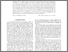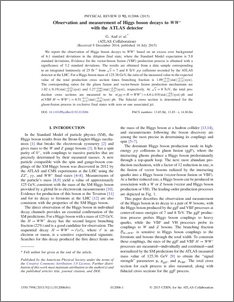# Observation and measurement of Higgs boson decays to WW∗ with the ATLAS detector

, The ATLAS collaboration (2015) Observation and measurement of Higgs boson decays to WW∗ with the ATLAS detector. Physical Review D, 92 (1). ISSN 1550-7998Preview
PDF (PhysRevD.92)
PhysRevD.92.pdf - Published Version

## Abstract

We report the observation of Higgs boson decays to WW ∗ based on an excess over background of 6.1 standard deviations in the dilepton final state, where the Standard Model expectation is 5.8 standard deviations. Evidence for the vector-boson fusion (VBF) production process is obtained with a significance of 3.2 standard deviations. The results are obtained from a data sample corresponding to an integrated luminosity of 25  fb −1 from s √ =7 and 8 TeV pp collisions recorded by the ATLAS detector at the LHC. For a Higgs boson mass of 125.36 GeV, the ratio of the measured value to the expected value of the total production cross section times branching fraction is 1.09 +0.16 −0.15 (stat) +0.17 −0.14 (syst) . The corresponding ratios for the gluon fusion and vector-boson fusion production mechanisms are 1.02±0.19(stat) +0.22 −0.18 (syst) and 1.27 +0.44 −0.40 (stat) +0.30 −0.21 (syst) , respectively. At s √ =8  TeV , the total production cross sections are measured to be σ(gg→H→WW ∗ )=4.6±0.9(stat) +0.8 −0.7 (syst)  pb and σ(VBF  H→WW ∗ )=0.51 +0.17 −0.15 (stat) +0.13 −0.08 (syst)  pb . The fiducial cross section is determined for the gluon-fusion process in exclusive final states with zero or one associated jet.

Item Type:
Journal Article
Journal or Publication Title:
Physical Review D